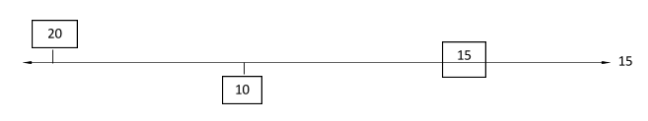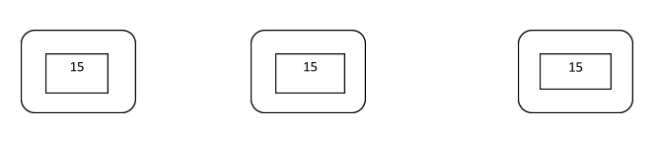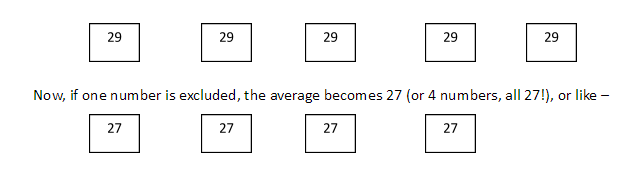# Averages

CONCEPT 1:

• Let’s understand the concept of averages with an example.

• Case I: If there are three rooms such that A has 20 students, B has 10 students and C has 15 students.

 20 10 15

Then the average number of students in the rooms is calculated as [(20+10+15)/3] = 15.

So, average refers to the sum of observations divided by the number of observations.

We got 15 as the average number of students in three rooms, what does that mean?  What is “15” trying to say? Let’s have a look.

We had three numbers (20,10,15) whose average is 15. Let’s say below is a line representing “15” (Average value).In this figure you can see that 20 is above the average, 10 is below the average and 15 is on the average line. Let us see how much deviation 20 & 10 has from the average number.

• 20 has +5 deviation (Positive deviation)

• 10 has -5 deviation (negative deviation)

Net deviation = Positive deviation + Negative deviation i.e. [(+5) + (-5)] =0

Oh! Did we get a zero? Isn’t that interesting? Yes, it is! Average of an observation is such a number which equals the net deviation of the whole observation to “zero”.

So, if there is no deviation in any of the numbers in an observation, then what does that mean? It means that the whole set of observation has same value. So, in above case when you got 15 as average number of students in three rooms, you can consider that each room has 15 students. This statement can also be represented as:Now, if I tell you to calculate the sum of observations in the above figure, what will you do?

Since you have to sum 15 three times so you will directly write 15*3=45 or you can rearrange this equation to 15=45/3. Can you observe this equation? This is exactly what you write as formula:

Average = Sum of observations/total number of observations

Now did you get an idea that if you know the concept, there is no need to memorize formula because ultimately these formulas were derived from the concepts. Also, average has many practical applications in our daily life, so if we understand the concept, we can utilize them

CONCEPT 2:

• Let’s understand approach towards solving a problem on average.

• Case 2: The average of five numbers is 29. If one number is excluded, the average becomes 27. What is the excluded number?

Solution: According to the question, the average of five numbers is 29. Now, using the concepts learnt above, Let’s suppose that value of all five numbers is 29 as shown in below figure.Now in the second arrangement, the numbers become 27. Originally, they were 29, now how do we calculate the lost/excluded number?

• Here is our observation: Looking at both figures we can clearly deduce that initially we had five boxes with value 29 and now we have four boxes with value 27, so we have clearly lost a number/box with value “29”. But Is 29 my excluded number? No, try to find other changes between two arrangements.

Can you see that apart from one missing box of value “29”, now every box has lost two units each. So, total number of units lost from all the four remaining boxes will be 4*2=8. So, now you can say that total number excluded/lost is 29+8=37.

• What is the textual method to solve above problem by using formula?

Average of five numbers =29, Let us consider five numbers be A, B, C, D, E. Now according to formula, Average= Sum of observations/Total number of observations. Applying formula, we can write
(A+B+C+D+E)/5=29
or
A+B+C+D+E= 145—Eq-1,
Since one number is excluded, we can write
(A+B+C+D)4=27
or
A+B+C+D=108— Eq-2.
Putting value of Eq-2 in Eq-1
we get A= (145-108) = 37.

Now, tell me, is it required to perform above mathematical operation to reach out to solution? Leave back your traditional approaches and start visualizing these problems. This will help you solve real life problems based on averages quickly without using pen & paper.

CONCEPT 3:

• How to visualize solutions to a problem related to average? Let’s solve a problem to learn this.

• Case 3: The average weight of the teacher and six students is 12 kg which is reduced by 5 kg if the weight of the teacher is excluded. How much does the teacher weigh?

Solution: Just visualize along. There are 7 people whose average weight is 12 kg, so we can consider that each of them weighs 12 kg. If the weight of teacher is excluded, average weight is reduced by 5 kg, so now there are 6 people who weigh 7 kg each. Now, let’s calculate total weight lost= [12 kg (teacher’s weight which was excluded)] + [30kg (5kgs each of 6 students)]. So, the excluded weight was 30+12=42 kg and whose weight were excluded? It was of the teacher. Thus, the teacher weighed 42 kg.

PROBLEMS ON AVERAGE

FOUNDATION

1. Find the average of the following set of scores 253, 124, 255, 534, 836, 375, 101, 443, 760.

1. 427

2. 413

3. 141

4. 490

5. None of these

2. What will be the average of even numbers between 11 to 63?

1. 37.5

2. 47

3. 42

4. 37

5. None of these

3. A, B, C, D & E are five consecutive even numbers. Average of A and E is 46. What is the largest number?

1. 52

2. 42

3. 50

4. 48

5. None of these

4. Find the average of first 25 multiples of 5.

1. 65

2. 60

3. 75

4. 80

5. None of these

5. 10 kg of rice priced at Rs. 12 per kg is mixed with 6 kg of rice priced at Rs. 16 per kg. Find the average price of the whole mixture?

1. Rs. 14 per kg

2. Rs. 14.50 per kg

3. Rs. 13 per kg

4. Rs. 13.50 per kg

5. None of these

MODERATE

1. The average speed of cyclist who covers first, second and third km at 20, 16 and 12 km/hr respectively (in km/hr) is:

1. 16.24

2. 16

3. 15.66

4. 15.32

5. None of these

1. Five years ago, the average age of P and Q was 15 years. Now, average of P, Q and R is 20 years. What would be the age of R after 10 years?

1. 35 year

2. 40 year

3. 30 year

4. 50 year

5. None of these

1. The average age of 24 boys and their teacher is 15 years. When the teacher’s age is excluded, the average age decreased by 1 year. The age of the teacher is?

1. 38 year

2. 39 year

3. 40 year

4. 41 year

5. None of these

1. The average salary of the entire staff in an office is Rs. 500 per day. The average salary of officer is Rs. 750 and that of non-officers is Rs. 250. If the number of officers is 15, then find number of non-officers in the office.

1. 25

2. 10

3. 15

4. 20

5. None of these

1. Three years ago, the average age of family of five members was 27 years. The present average age is also 27 years after the inclusion of a child into family. Find the age of the child.

1. 16 years

2. 12 years

3. 24 years

4. 20 years

5. None of these

HOTS -HIGH ORDER THINKING SKILLS

1. The average weight of A, B and C is 84 kgs. If D joins, the average weight now is 80 kgs. If another person E who is 3 kgs heavier than D replaces A then the average weight of B, C, D and E becomes 79 kgs. What is the weight of A?

1. 70 kg

2. 75 kg

3. 65 kg

4. 55 kg

5. None of these

1. A batsman has an average of 30 runs in his 42 innings. The difference between his maximum and minimum score is 100. If these two innings are removed his average for 40 innings are removed his average for 40 innings comes down to 28. What is his minimum score?

1. 20

2. 30

3. 45

4. 40

5. None of these

1. There were 35 students in a hostel. If the number of students increase by 7, the expenses of mess increase by Rs. 42 per day while the average expenditure per head decreases by Rs. 1. Find the original expenditure of the mess.

1. Rs. 420

2. Rs. 520

3. Rs. 450

4. Rs. 550

5. None of these

1. The average salary per head of all the workers in a company in Rs. 95. The average salary of 15 officers is RS. 525 and the average salary per head of the rest is Rs. 85. Find the total number of workers in the workshop.

1. 660

2. 580

3. 650

4. 460

5. None of these

1. The average age of a group of persons going for a picnic is 16.75 years. 20 new persons with an average age of 13.25 years join the group on the spot due to which the average of group becomes 15 years. Find the number of persons initially going for the picnic?

1. 24

2. 20

3. 15

4. 18

5. None of these

SOLUTIONS

FOUNDATION

1. (e)
Average = (253+124+255+534+836+375+101+443+760)/9
= 409

2. (d)
Total even number = (63 – 11)/2
= 52/2
= 26
Sum of the even number between 11 to 63
= 26/2 [2×12+(26-1) ×2]
= 13 [24+50]
= 13 × 74
= 962
Average of even number between 11 to 63
= 962/26
= 37

3. (c)
Let A is x
.’. Then E = x + 8
According to the question
(x + x + 8)/2 = 46
» 2x = 48
» x = 42
largest number is 42 + 8 = 50

4. (a)
Sum of first 25 multiples of 5
= 5 (1+2+3….25)
= 5 × {(25 × 26)/2}
= 1625
Average = 1625/25 = 65

5. (d)
Total price of 10 kg Rice = 12 × 10
= 120
Total price of 6 kg Rice = 16 × 6
= 96
.’. Average = (120 + 96)/16
= 216/16
= 13.50 kg

MODERATE

1. (d)
Let the average speed of a cyclist = x
Total distance = 3 km
.’. 1/20 + 1/16 + 1/12 = 3/x
» (12 + 15 + 20)/240 = 3/x
» 47/240 = 3/x
» x = 15.32 km/hr

2. (c)
Total present age of P and Q = 15 × 2 + 10 = 40 years
Total present age of P, Q and R = 20 × 3 = 60 years
P + R + Q = 60 years
(present age) R = 20 years
R age after 10 year = 20 + 10 = 30 years

3. (b)
Total age of 24 boys and teacher = (24  + 1) × 15 = 375 years
Total age of all boys excluding teacher = 24 × (15 – 1) = 336 years
Age of teacher = 375 – 336 = 39 years
SHORTCUT: Age of teacher = 15 + 24 × 1 = 39 years

4. (c)
Let the total no. of non-officer staff in an office = x
According to the question
750 × 15 + 250x = 500 (x + 15)
» 500x – 250x = 11250 – 7500
» 250x = 3750
» x = 3750/250 = 15
Hence no. of non-officer staff = 15

5. (b)
Total present age of family of 5 members
= 27 × 5 + 3 × 5 = 135 + 15 = 150 years
Total present age of family including child
= 27 × 6 = 162 years
Age of a child = 162 – 150 = 12 years.

HOTS- HIGH ORDER THINKING SKILLS

1. (b)
Total weight of A + B + C = 84 × 3 = 252 Kgs
A + B + C + D = 80 × 4 = 320 Kgs
D = 68, E = 68 + 3 = 71 kgs
B + C + D + E = 79 × 4 = 316 kgs
B + C + D = 316 – 71 = 245 kgs
A = 320 – 245 = 75 kgs

2. (a)
Total runs of 42 innings = 42 × 30 = 1260
Total run excluding min and max inning
= 40 × 28 = 1120
max + min = 1260 – 1120 = 140
max – min = 100
On solving (i) and (ii)
max = 120, min = 20
Hence, minimum score is 20.

3. (a)
Let the average expenditure of a man = x Rs.
.’. According to the question
.’. 35 × x + 42 = 42 (x – 1)
.’. 7x = 84
» x = 12 Rs.
Original expenditure = 35 × 12 = 420 Rs.

4. (a)
Let the total number of workers = x
.’. 15 × 525 + (x – 15) 85 = x ×95
.’. 15 × 525 – 15 × 85 = 10x
.’. 7875 – 1275 = 10x
» 10x = 6600
» x = 660

(b)
Let the person initially going for picnic is x
.’. 16.75 × x + 20 × 13.25 = 15 (x + 20)
1.75x = 15 × 20 – 20 × 13.25
1.75x = 35
x = 20